# Cyclic colormaps comparison

I started this post looking for a diffusion map on Python, that I didn't find. Then I continued following an example on manifold learning by Jake Vanderplas on a different dataset. It worked nicely,but the colormap used is Spectral, that is divergent. This made me think about using a cyclic colormap, and ended up in this StackOverflow question. And I decided to compare some cyclic colormaps.

I picked up colormaps from different sources

• phase

• hue_L60

• erdc_iceFire

• nic_Edge

• colorwheel

• cyclic_mrybm_35_75_c68

• cyclic_mygbm_30_95_c78

and, of course, hsv. You can download the colormaps in text format from here.

## Comparison

For all the examples below the following imports are done:

from __future__ import division, print_function
import numpy as np
import matplotlib.pyplot as plt
from matplotlib.colors import LinearSegmentedColormap
from colorspacious import cspace_convert


### Color map test image

Peter Kovesi proposed a way to compare cyclic colormaps on a paper on 2015. It consists of a spiral ramp with an undulation. In polar coordinates it reads

\begin{equation*} c = (A \rho^p \sin(n \theta) + \theta)\, \mathrm{mod}\, 2\pi \end{equation*}

with $A$ the amplitude of the oscilation, $\rho$ the normalized radius in [0, 1], $p$ a positive number, and $n$ the number of cycles.

And the following function creates the grid in Python

def circle_sine_ramp(r_max=1, r_min=0.3, amp=np.pi/5, cycles=50,
power=2, nr=50, ntheta=1025):
r, t = np.mgrid[r_min:r_max:nr*1j, 0:2*np.pi:ntheta*1j]
r_norm = (r - r_min)/(r_max - r_min)
vals = amp * r_norm**power * np.sin(cycles*t) + t
vals = np.mod(vals, 2*np.pi)
return t, r, vals


The following is the result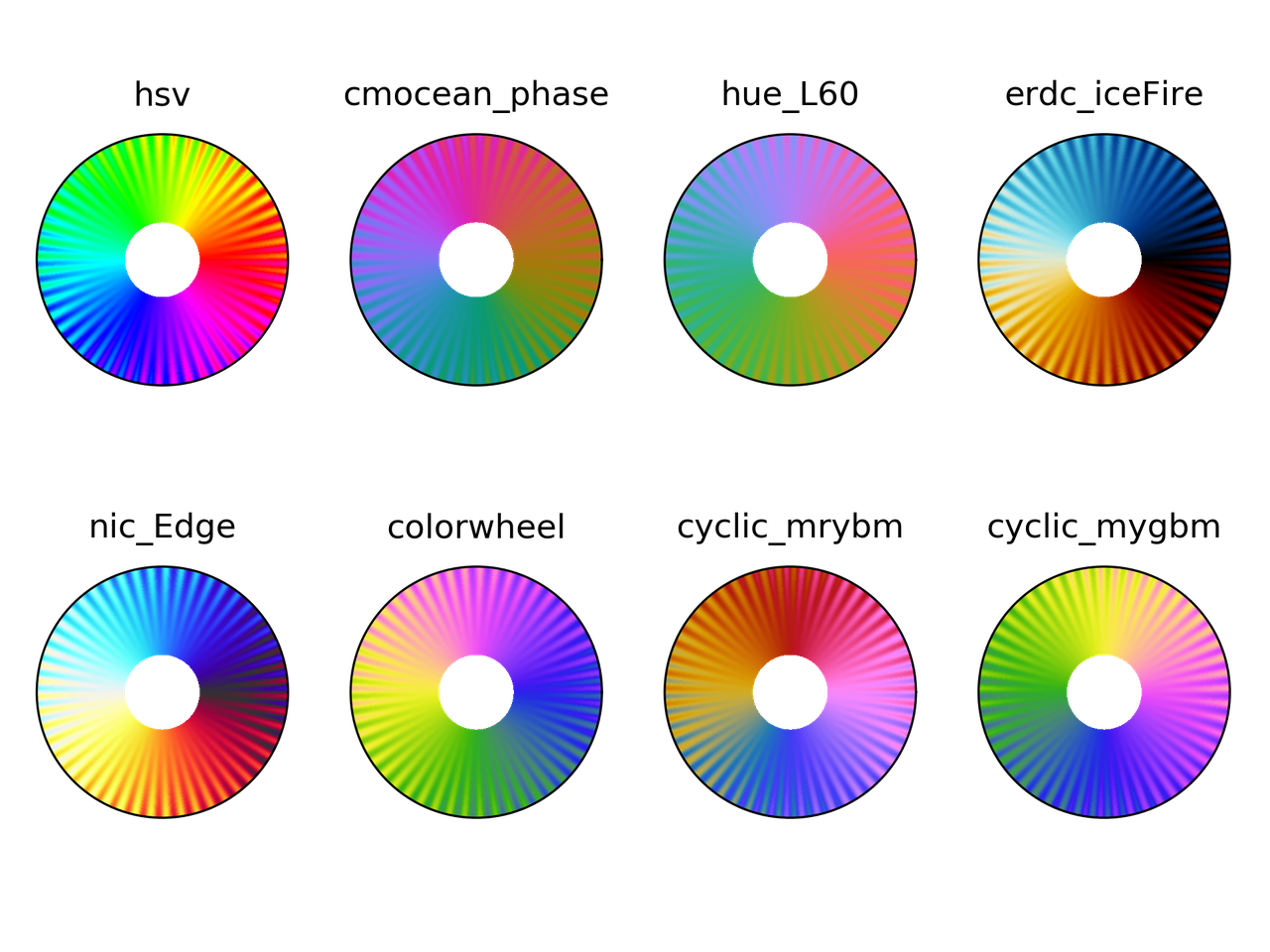### Colorblindness test

t, r, vals = circle_sine_ramp(cycles=0)
cmaps = ["hsv",
"cmocean_phase",
"hue_L60",
"erdc_iceFire",
"nic_Edge",
"colorwheel",
"cyclic_mrybm",
"cyclic_mygbm"]
severity = [0, 50, 50, 50]
for colormap in cmaps:
fig = plt.figure()
for cont in range(4):
cvd_space = {"name": "sRGB1+CVD",
"cvd_type": cvd_type[cont],
"severity": severity[cont]}
data2 = cspace_convert(data, cvd_space, "sRGB1")
data2 = np.clip(data2, 0, 1)
cmap = LinearSegmentedColormap.from_list('my_colormap', data2)
ax = plt.subplot(2, 2, 1 + cont, projection="polar")
ax.pcolormesh(t, r, vals, cmap=cmap)
ax.set_xticks([])
ax.set_yticks([])
plt.suptitle(colormap)
plt.tight_layout()
plt.savefig(colormap + ".png", dpi=300)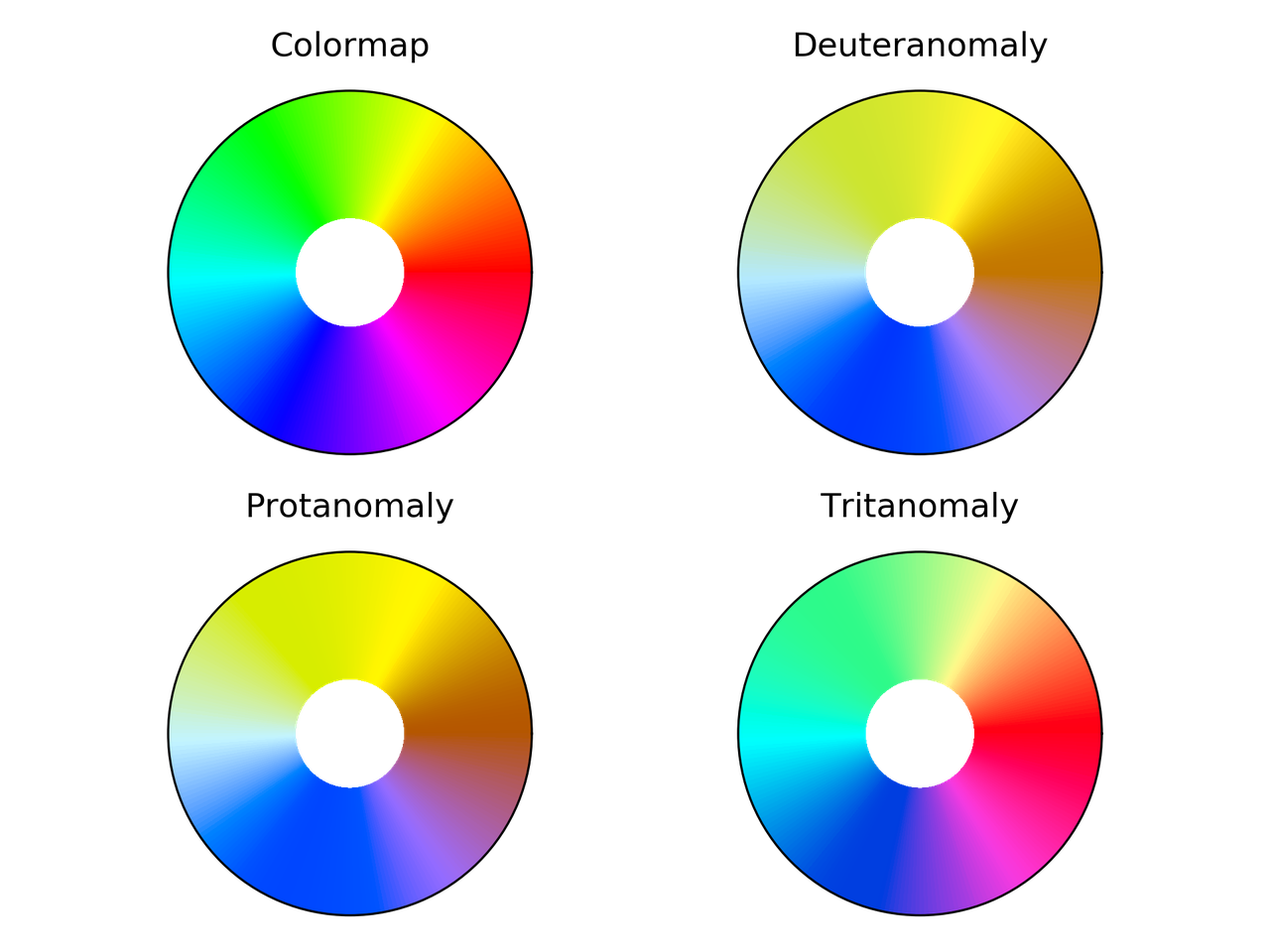hsv colormap comparison for different color vision deficiencies.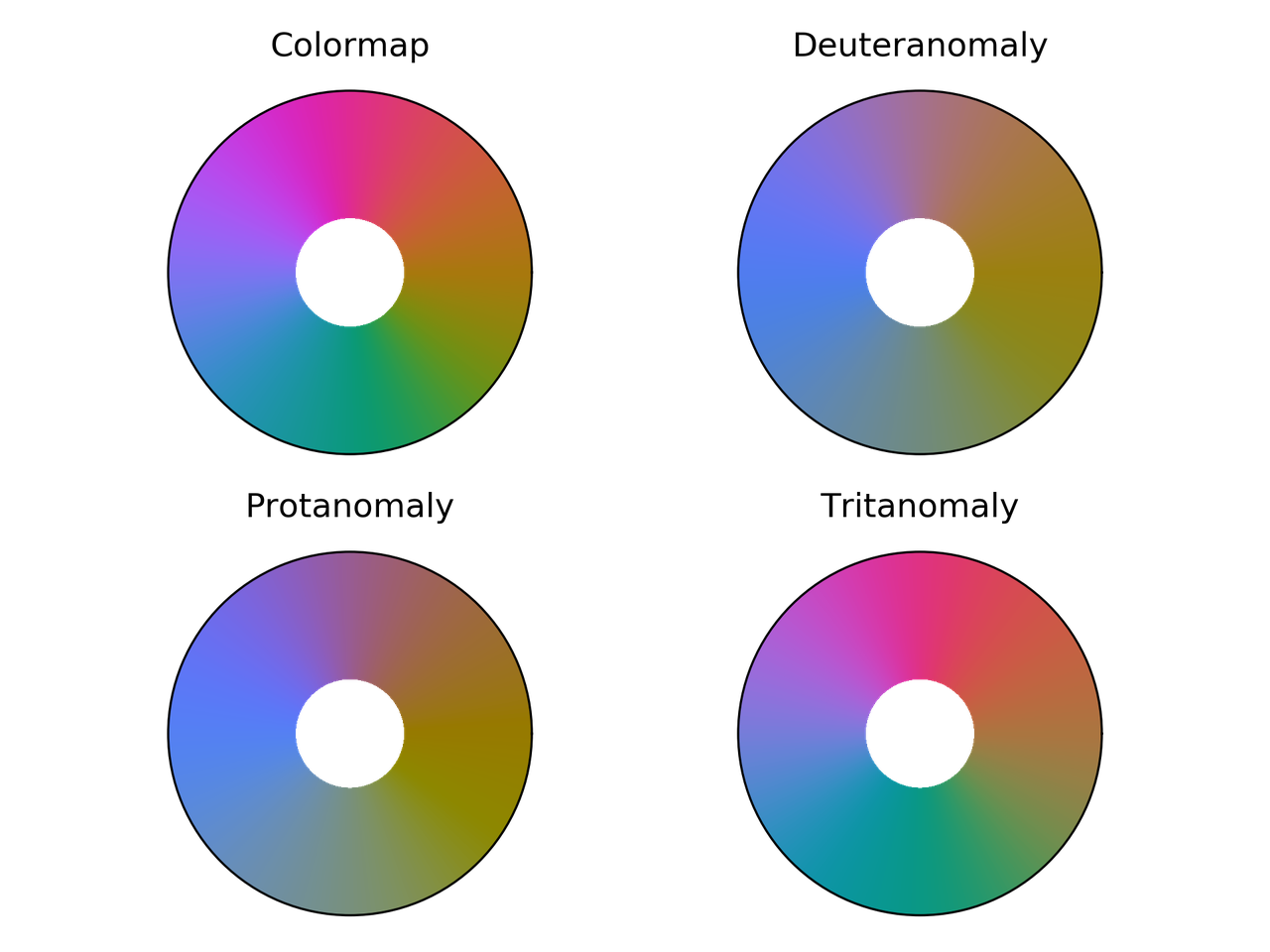Phase colormap comparison for different color vision deficiencies.hue_L60 colormap comparison for different color vision deficiencies.erdc_iceFire colormap comparison for different color vision deficiencies.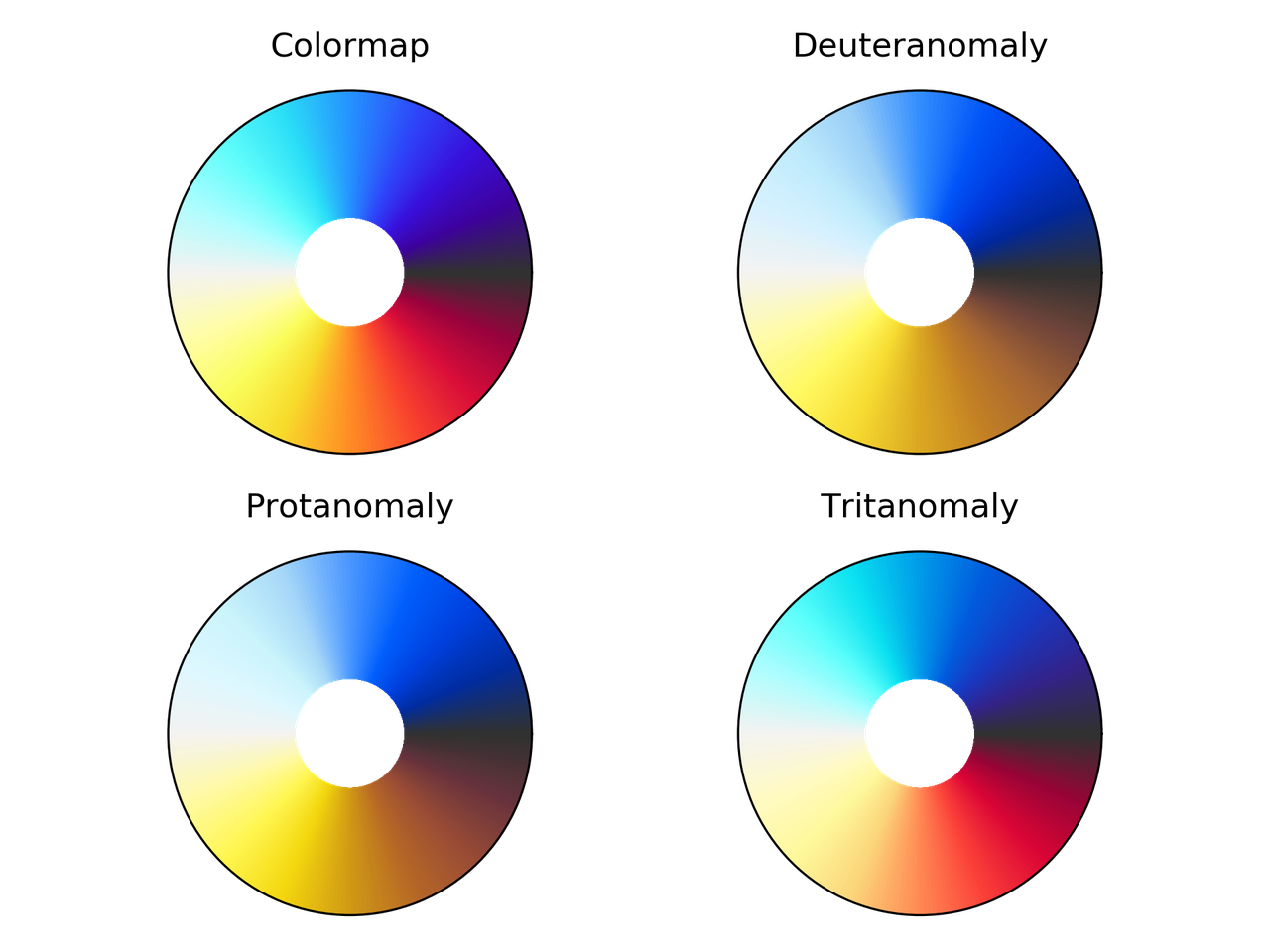nic_Edge colormap comparison for different color vision deficiencies.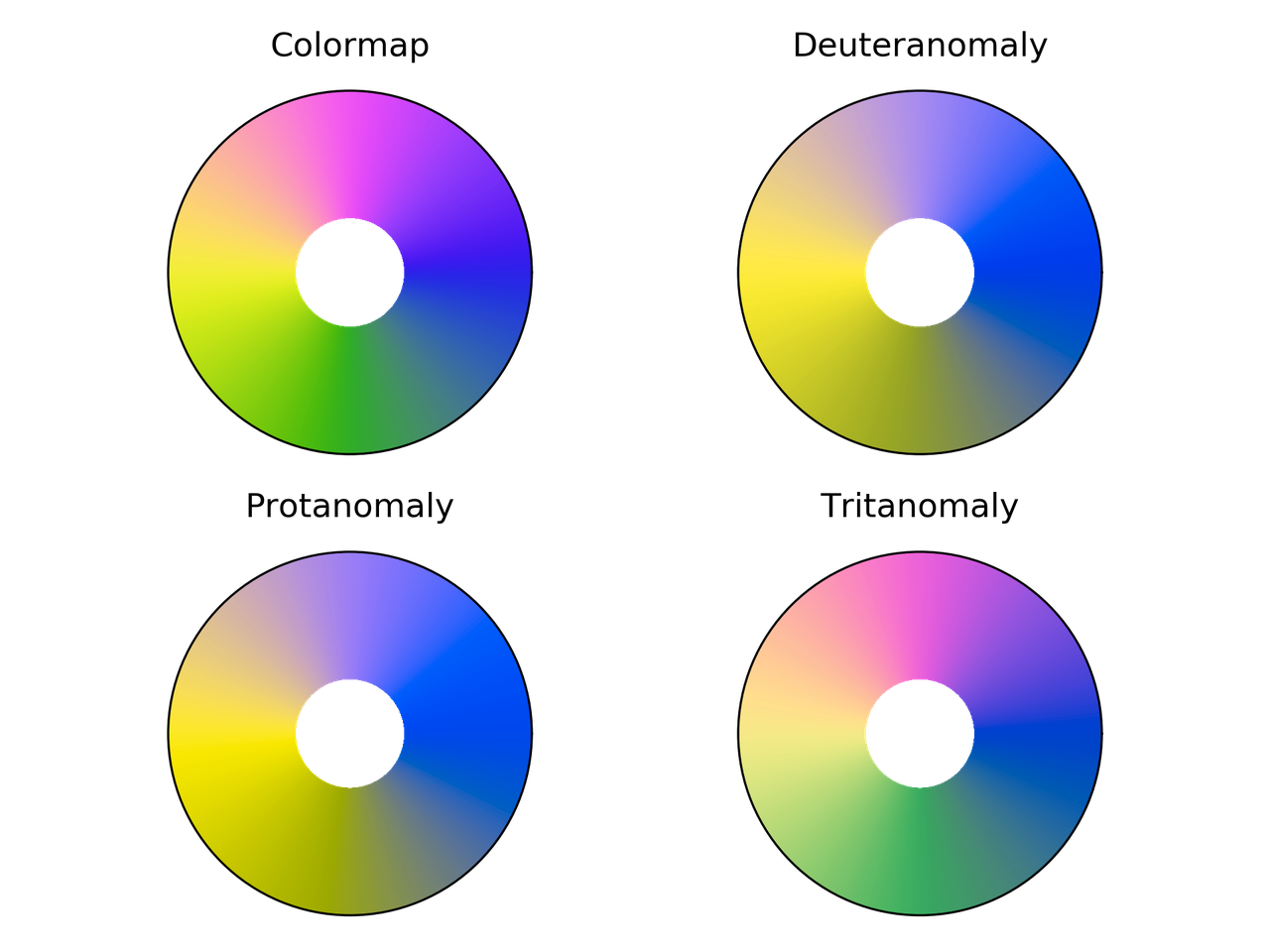Colorwheel colormap comparison for different color vision deficiencies.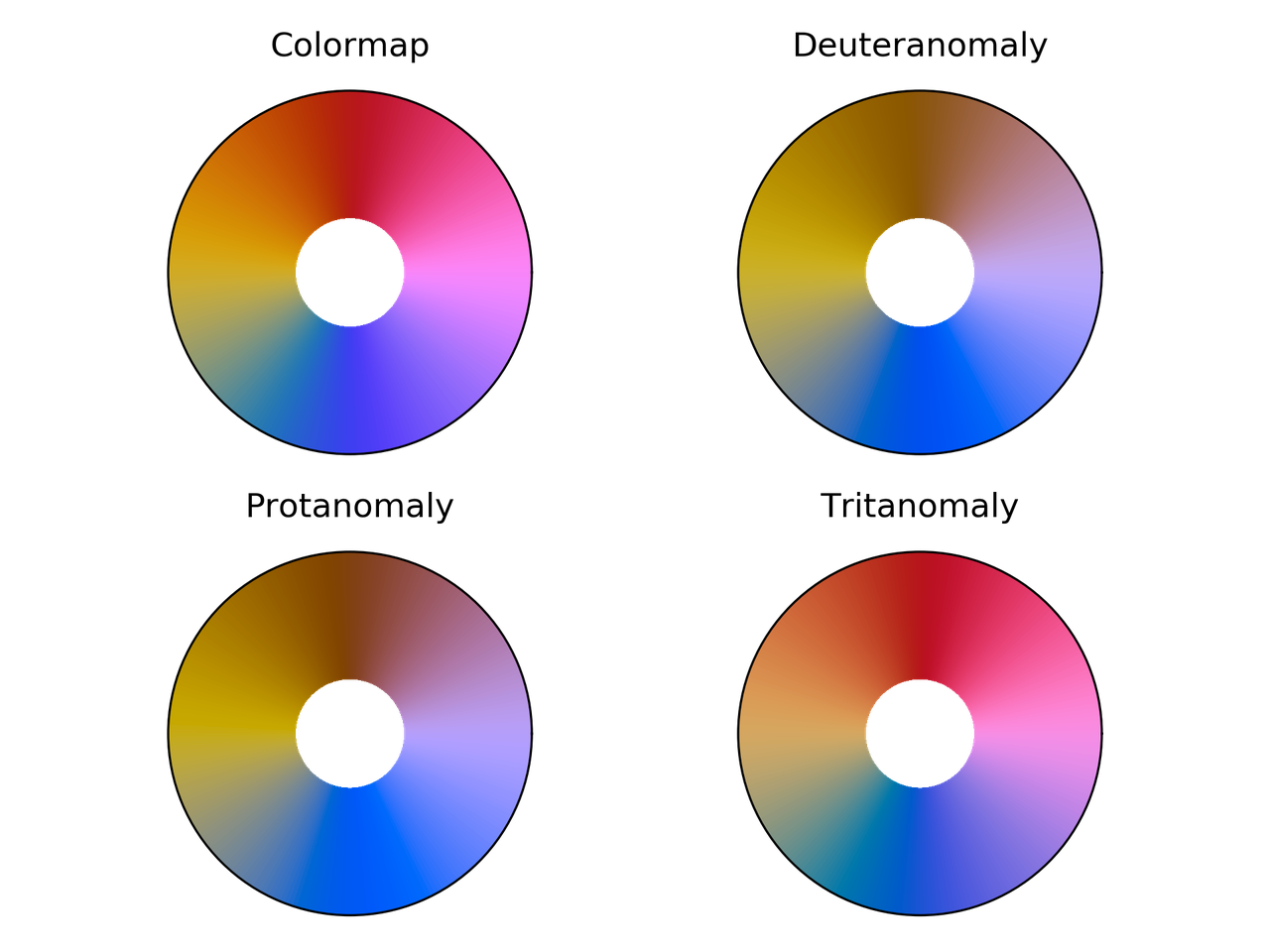Cyclic_mrybm colormap comparison for different color vision deficiencies.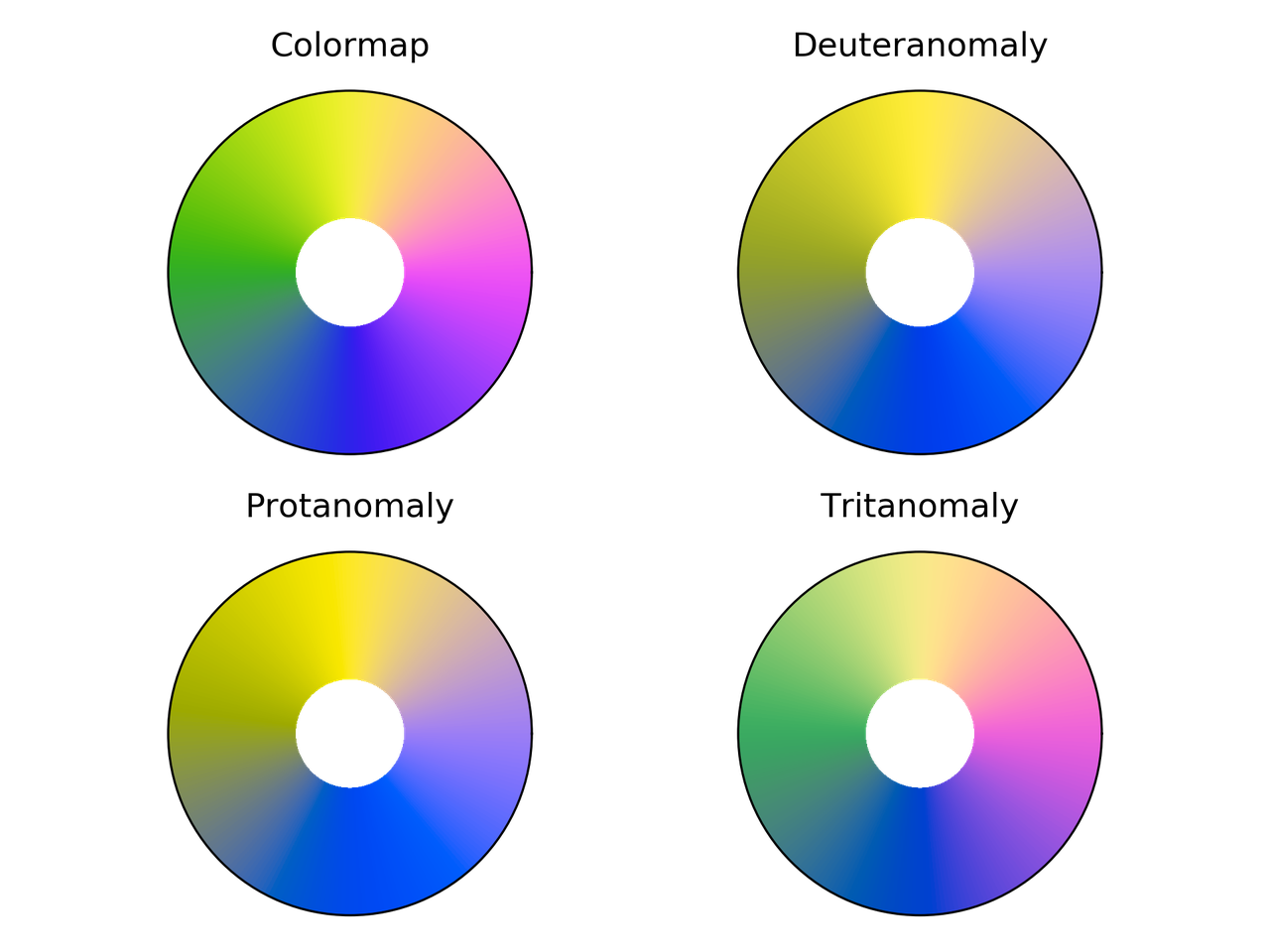Cyclic_mygbm colormap comparison for different color vision deficiencies.

## Randomly generated cyclic colormaps

What if we generate some random colormaps that are cyclic? How would they look like?

Following are the snippet and resulting colormaps.

from __future__ import division, print_function
import numpy as np
from mpl_toolkits.mplot3d import Axes3D
import matplotlib.pyplot as plt
from matplotlib.colors import LinearSegmentedColormap

# Next line to silence pyflakes. This import is needed.
Axes3D

plt.close("all")
fig = plt.figure()
fig2 = plt.figure()
nx = 4
ny = 4
azimuths = np.arange(0, 361, 1)
zeniths = np.arange(30, 70, 1)
values = azimuths * np.ones((30, 361))
for cont in range(nx * ny):
np.random.seed(seed=cont)
mat = np.random.rand(3, 3)
rot_mat, _ = np.linalg.qr(mat)
t = np.linspace(0, 2*np.pi, 256)
z = 0.0*np.cos(t)
X = np.vstack((x, y, z))
X = rot_mat.dot(X) + center

ax = fig.add_subplot(ny, nx, 1 + cont, projection='polar')
cmap = LinearSegmentedColormap.from_list('my_colormap', X.T)
ax.pcolormesh(azimuths*np.pi/180.0, zeniths, values, cmap=cmap)
ax.set_xticks([])
ax.set_yticks([])

ax2 = fig2.add_subplot(ny, nx, 1 + cont, projection='3d')
ax2.plot(X[0, :], X[1, :], X[2, :])
ax2.set_xlim(0, 1)
ax2.set_ylim(0, 1)
ax2.set_zlim(0, 1)
ax2.view_init(30, -60)
ax2.set_xticks([0, 0.5, 1.0])
ax2.set_yticks([0, 0.5, 1.0])
ax2.set_zticks([0, 0.5, 1.0])
ax2.set_xticklabels([])
ax2.set_yticklabels([])
ax2.set_zticklabels([])

fig.savefig("random_cmaps.png", dpi=300, transparent=True)
fig2.savefig("random_cmaps_traj.svg", transparent=True)16 randomly generated colormaps.Trajectories in RGB space for the randomly generated colormaps.

A good idea would be to take these colormaps and optimize some perceptual parameters such as lightness to get some usable ones.

## Conclusions

I probably would use phase, colorwheel, or mrybm in the future.Initial image using phase.Initial image using colorwheel.Initial image using mrybm.